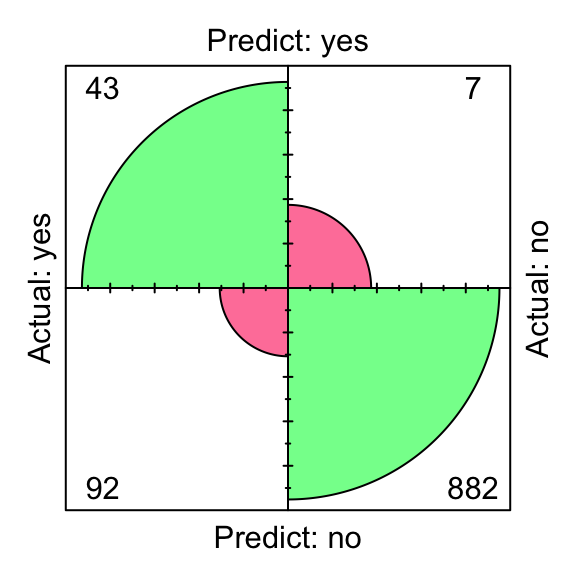The `liver` package contains a collection of helper functions that make various techniques from data science more user-friendly for non-experts.

Here is an example to show how to use the functionality of the package by using the churn dataset which is available in the package.

``````data( churn )

str( churn )
'data.frame': 5000 obs. of  20 variables:
\$ state         : Factor w/ 51 levels "AK","AL","AR",..: 17 36 32 36 37 2 20 25 19 50 ...
\$ area.code     : Factor w/ 3 levels "area_code_408",..: 2 2 2 1 2 3 3 2 1 2 ...
\$ account.length: int  128 107 137 84 75 118 121 147 117 141 ...
\$ voice.plan    : Factor w/ 2 levels "yes","no": 1 1 2 2 2 2 1 2 2 1 ...
\$ voice.messages: int  25 26 0 0 0 0 24 0 0 37 ...
\$ intl.plan     : Factor w/ 2 levels "yes","no": 2 2 2 1 1 1 2 1 2 1 ...
\$ intl.mins     : num  10 13.7 12.2 6.6 10.1 6.3 7.5 7.1 8.7 11.2 ...
\$ intl.calls    : int  3 3 5 7 3 6 7 6 4 5 ...
\$ intl.charge   : num  2.7 3.7 3.29 1.78 2.73 1.7 2.03 1.92 2.35 3.02 ...
\$ day.mins      : num  265 162 243 299 167 ...
\$ day.calls     : int  110 123 114 71 113 98 88 79 97 84 ...
\$ day.charge    : num  45.1 27.5 41.4 50.9 28.3 ...
\$ eve.mins      : num  197.4 195.5 121.2 61.9 148.3 ...
\$ eve.calls     : int  99 103 110 88 122 101 108 94 80 111 ...
\$ eve.charge    : num  16.78 16.62 10.3 5.26 12.61 ...
\$ night.mins    : num  245 254 163 197 187 ...
\$ night.calls   : int  91 103 104 89 121 118 118 96 90 97 ...
\$ night.charge  : num  11.01 11.45 7.32 8.86 8.41 ...
\$ customer.calls: int  1 1 0 2 3 0 3 0 1 0 ...
\$ churn         : Factor w/ 2 levels "yes","no": 2 2 2 2 2 2 2 2 2 2 ...``````

It shows that the ‘churn’ dataset as a `data.frame` has 20 variables and 5000 observations.

# Partitioning the dataset

We partition the churn dataset randomly into two groups: train set (80%) and test set (20%). Here, we use the `partition` function from the liver package:

``````set.seed( 5 )

data_sets = partition( data = churn, prob = c( 0.8, 0.2 ) )

train_set = data_sets \$ part1
test_set  = data_sets \$ part2

actual_test  = test_set \$ churn``````

# Classification by kNN algorithm

The churn dataset has 19 predictors along with the target variable `churn`. Here we use the following predictors:

`account.length`, `voice.plan`, `voice.messages`, `intl.plan`, `intl.mins`, `day.mins`, `eve.mins`, `night.mins`, and `customer.calls`.

First, based on the above predictors, find the k-nearest neighbor for the test set, based on the training dataset, for the k = 8 as follows

``````formula = churn ~ account.length + voice.plan + voice.messages + intl.plan + intl.mins +
day.mins + eve.mins + night.mins + customer.calls

predict_knn = kNN( formula, train = train_set, test = test_set, k = 8 )``````

To report Confusion Matrix:

``````conf.mat( predict_knn, actual_test )
Actual
Predict yes  no
yes  43   7
no   92 882

conf.mat.plot( predict_knn, actual_test )``````To report Mean Squared Error (MSE):

``````mse( predict_knn, actual_test )
 0.09667969``````

# Classification by kNN algorithm with data transformation

The predictors that we used in the previous part, do not have the same scale. For example, variable `day.mins` change between 0 and 351.5, whereas variable `voice.plan` is binary. In this case, the values of variable `day.mins` will overwhelm the contribution of `voice.plan`. To avoid this situation we use normalization. So, we use min-max normalization and transfer the predictors as follows:

``predict_knn_trans = kNN( formula, train = train_set, test = test_set, k = 8, transform = "minmax" )``

To report Confusion Matrix:

``````conf.mat.plot( predict_knn_trans, actual_test )

conf.mat.plot( predict_knn, actual_test )``````